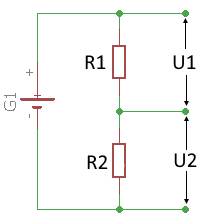# Voltage divider

Online calculator for calculating the values on an unloaded voltage divider

## Unbelasteter Spannungsteiler

On this page you can calculate the voltages and resistances of a voltage divider. To calculate it, two voltages and one resistance or two resistances and one voltage must be known.

To calculate, enter three values in one of the following combinations:

• 2 voltage values and 1 resistance value
• 1 voltage value and 2 resistance values

 Enter 1 or 2 voltages Total voltage mV V Voltage U1 mV V Voltage U2 mV V Enter 1 or 2 resistors Total resistance mΩ Ω kΩ MΩ Resistor R1 mΩ Ω kΩ MΩ Resistor R2 mΩ Ω kΩ MΩ Decimal places 0 1 2 3 4 6 8 10 Results Total voltage Voltage U1 Voltage U2 Total resistance Resistor R1 Resistor R2## Voltage divider formulas

The voltage divider is a series circuit of resistors that divide an electrical voltage. The ratio of the partial voltages corresponds to the ratio of the resistances. So for the calculation of the partial voltage $$\displaystyle U_2$$ at $$\displaystyle R_2$$ can be calculated:

$$\displaystyle U_2=\frac{U_{ges}} {R_{ges}} · R_2$$

Alternatively, the partial voltage $$\displaystyle U_2$$ at $$\displaystyle R_2$$ can be calculated using the current $$\displaystyle I$$. To do this, the total resistance is first calculated as follows:

$$\displaystyle R_{ges}=R_1+R_2$$

Assuming the total voltage is known, the current $$\displaystyle I$$ can be determined according to Ohm's law:

$$\displaystyle I=\frac{U_{ges}} {R_{ges}}$$

The partial voltage at $$\displaystyle R_2$$ can be calculated:

$$\displaystyle U_2=R_2 · I$$

Resistor $$\displaystyle R_1$$

$$\displaystyle R_1=\frac{U_1 · R_2}{U_2}\,=\,\frac{U_1 · R_2}{U_G-U_1}$$

Resistor $$\displaystyle R_2$$

$$\displaystyle R_2=\frac{U_2 · R_1}{U_1}\,=\,\frac{R_1 (U_G-U_1)}{U_1}$$